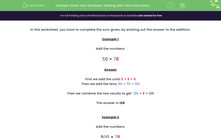# Use Partitioning to Add Numbers

In this worksheet, students will improve their mental addition by adding two and three-digit numbers that include tens and hundreds.Key stage:  KS 2

Curriculum topic:   Number: Addition and Subtraction

Curriculum subtopic:   Use Mental Addition/Subtraction to 1000

Difficulty level:#### Worksheet Overview

In this activity, we will be adding numbers using partitioning into tens and ones.

In a two-digit number, the digit on the left represents the number of tens and the digit on the right represents the number of ones.

Let's look at 48 as an example.

48

48 has 4 tens and 8 ones.

When adding two two-digit numbers, we can partition them both into tens and ones to make the calculation easier.

To add the numbers 50 and 78, we partition them and add the ones, then the tens.

50 + 78

First we add the units 0 + 8 = 8

Then we add the tens, 50 + 70 = 120

Then we combine the two results to get  120 + 8 = 128

The total is 128

Now let's try another example together. This one has a three-digit number to consider.

Example

500 + 78

First, we add the units 0 + 8 = 8

Then we add the tens, 00 + 70 = 70

Then we add the hundreds, 500 + 000 = 500

Then we combine the three results to get   500 + 70 + 8 = 578

Now it's your turn to try some similar questions.### What is EdPlace?

We're your National Curriculum aligned online education content provider helping each child succeed in English, maths and science from year 1 to GCSE. With an EdPlace account you’ll be able to track and measure progress, helping each child achieve their best. We build confidence and attainment by personalising each child’s learning at a level that suits them.

Get started